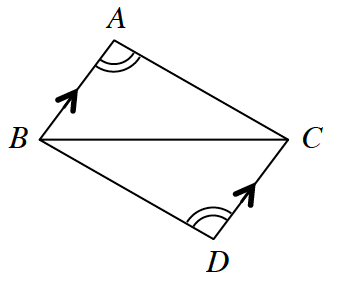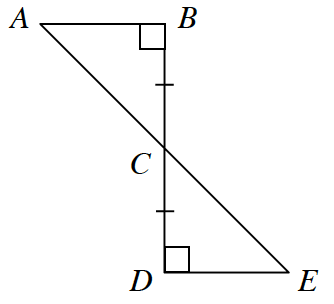### Home > CCG > Chapter 8 > Lesson 8.2.2 > Problem8-88

8-88.

For each pair of triangles below, decide if the triangles are congruent. If the triangles are congruent:

• State which triangle congruence property proves that the triangles are congruent.

• Write a congruence statement (such as $\Delta ABC \cong \Delta \text{___}$ ).

1.What parts of these triangles makes them congruent? Do they share any parts?

AAS $\cong$, $\Delta ABC \cong \Delta DCB$

1.Use the same method as part (a), but use a different congruence property.
Are there unmarked corresponding parts that are congruent?